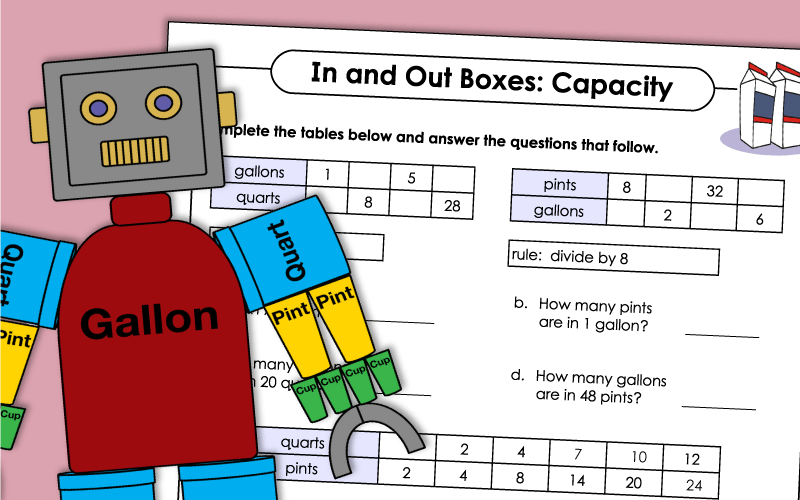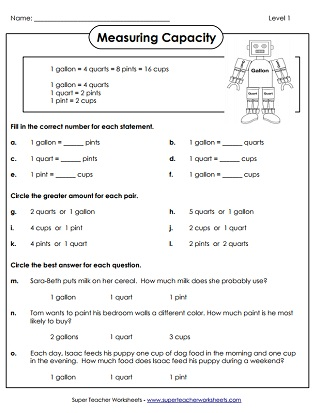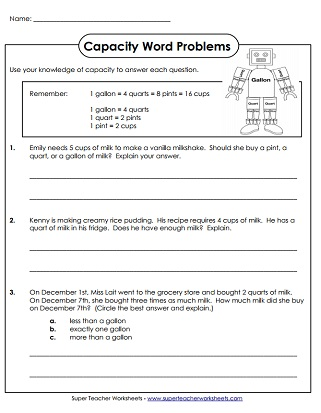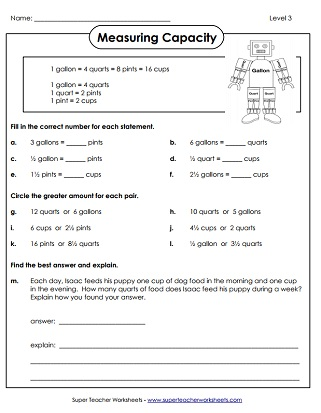# Capacity Worksheets

These printable measurement worksheets for measuring capacity or volume.  These worksheets use standard, customary units: gallons, quarts, pints, and cups.Use the picture of GallonBot to answer the questions about gallons, quarts, pints, and cups.
Assemble a large GallonBot figure; Sometimes referred to as Gallon Man, Measurement Man, or Mr. Gallon.
A single-page GallonBot picture that students can color and use as a reference.
Students use their knowledge of capacity to answer the questions.
Practice converting between cups, pints, quarts, and gallons. The worksheet includes two word problems.
This worksheet has a variety of types of questions involving converting pints, cups, quarts, and gallons.
Measurement bookmarks for reference.  Includes capacity and linear measurement.
Students are required to calculate equal amounts, compare capacity amounts, and solve a word problem.
Same as above, but a little more difficult.  Students are required to compare multiple gallons, quarts, pints, and cups.
Advanced capacity questions require students to calculate to the nearest half units.
Linear Measurement (Feet, Inches)

Measure objects and pictures in feet and inches

Metric Capacity - (Liters and Milliliters)

Measure capacity (volume) in metric units, milliliters and liters.

Measurement Worksheets

Worksheets for linear measurement, capacity, temperature, mass, and volume.

## Worksheet ImagesMy Account
Site Information